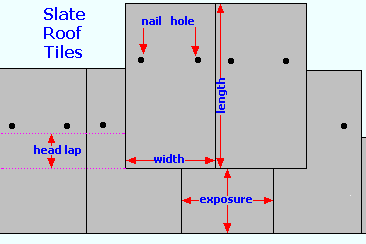Spike's Calculators

# Slate Roof Tiles - MetricCalculate the number of Slate tiles needed based on the square metres of the roof. Slate roofing tiles come in various sizes.### Slate Roof Tiles - Meters

Roof Area
Tile Length cm
Tile Width cm
Waste %

#### Results:

 Tile Exposure m² Number of Tiles per Square Metre # Tiles Needed tiles Waste Percentage Adds # of tiles Total # of Tiles Needed tiles

#### Calculation

1. enter the area of the roof in square metres
2. slate tile head lap in centimetres
3. tile length in centimetres
4. tile width in centimetres
5. waste percentage preset @ 12%

#### Results

1. exposure of the tile in square metres
2. number of tiles needed to cover one square metre
3. amount of tiles needed based on square metres
4. number of tiles added for waste
5. total number of tiles needed